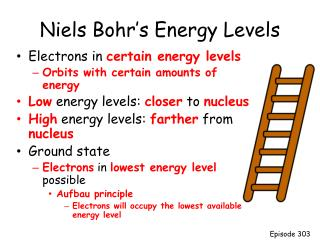DownloadDownload PresentationNiels Bohr’s Energy Levels

# Niels Bohr’s Energy Levels

Download Presentation## Niels Bohr’s Energy Levels

- - - - - - - - - - - - - - - - - - - - - - - - - - - E N D - - - - - - - - - - - - - - - - - - - - - - - - - - -
##### Presentation Transcript

1. Niels Bohr’s Energy Levels • Electrons in certain energy levels • Orbits with certain amounts of energy • Low energy levels: closer to nucleus • High energy levels: farther from nucleus • Ground state • Electrons in lowest energy level possible • Aufbau principle • Electrons will occupy the lowest available energy level Episode 303

2. Excited Atom • Atom has absorbed energy • Quanta – Energy required to excite an electron • Excited state is unstable • Atom soon emits same amount of absorbed energy • Energy seen as visible light • Photon – particle with no mass containing a quanta of energy • COLOR Episode 303

3. Wave Description of Light • Wavelength (λ) • Distance between corresponding points on adjacent waves • Frequency(f or γ) • The number of waves passing a given point in a given time • Hertz • C = λf • C = 3.0 x 108 m/s : speed of light Episode 303

4. Electromagnetic Spectrum http://education.jlab.org/frost/speed_of_light.html • What is the frequency of light if the wavelength is 6.0 x 10-7 m? C = λf • C = 3.0 x 108 m/s : speed of light • 3.0 x 108 m/s = (6.0 x10-7 m) f • 3.0 x 108 m/s • = f • 6.0 x10-7 m • 5.0 x 1014/s = f Episode 303

5. Electromagnetic Spectrum • What is the wavelength of light if its frequency is 5.0 x 1014 Hertz? C = λf • C = 3.0 x 108 m/s : speed of light • 3.0 x 108 m/s = λ(5.0 x 1014/s) • 3.0 x 108 m/s • = λ • 5.0 x 1014/s • 6.0 x 10-7 m = λ Episode 303

6. Particle Description of Light • Energy exists as particles called quanta • Photoelectric Effect • Metal emits electrons when light strikes the metal • E =hf • h is Plank’s Constant • 6.626 x 10-34 Js Episode 303

7. Electromagnetic Spectrum • How much energy does light contain if its frequency is 5.0 x 1014 Hertz? E = hf • h = 6.626 x 10-34 Js : Plank’s Constant • E= (6.626 x 10-34Js)(5.0 x 1014/s) • E = 3.3 x 10-19 J Episode 303

8. The Modern View of Light • Light has a dual nature • Light may behave as a wave • Light may behave as a stream of particles called quanta or photons Episode 303

9. Spectroscopy • Spectral lines represent energy released as electrons return to lower energy levels • Spectral lines identify an element • Called the Bright Line Spectrum of an Element Episode 303

10. Orbital http://www.youtube.com/watch?v=rNM21emkMJo&feature=related • Region of space where an electron is likely to be found • Heisenberg Uncertainty Principle • Impossible to determine location and velocity of a particle Episode 303